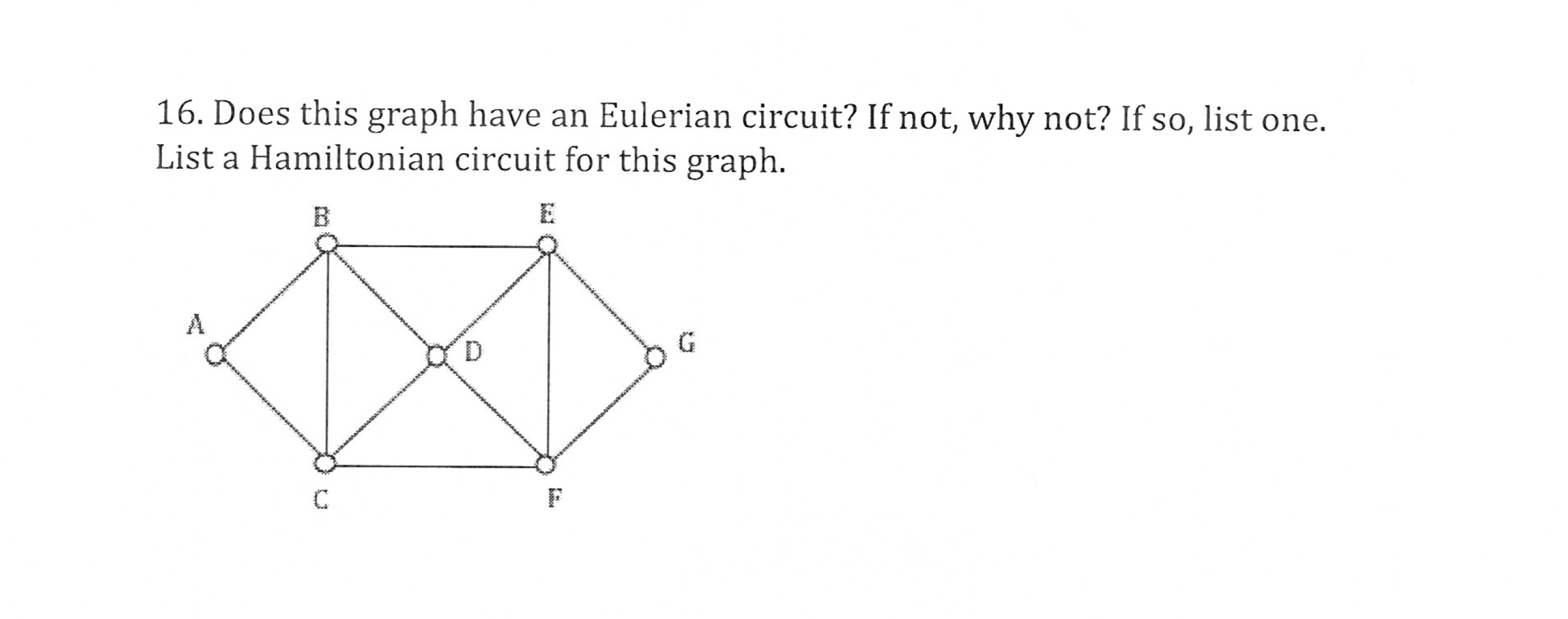# 16. Does this graph have an Eulerian circuit? If not, why not? If so, list one.List a Hamiltonian circuit for this graph

Question
15 viewshelp_outlineImage Transcriptionclose16. Does this graph have an Eulerian circuit? If not, why not? If so, list one. List a Hamiltonian circuit for this graph fullscreen
check_circle

Step 1

To investigate the given graph for the required circuit  properties

Step 2

An Eulerian circuit in a graph is a closed path (starting and ending at the same vertex) , traversing theough each edge exactly once. A necessary and sufficient condition for an Euler circuit to exist is that the degree of each vertex is even, which is the case here.

Step 3

In the given graph, degrees of all vertices are even So , an Euler circuit must exist. For example, traverse the graph s...

### Want to see the full answer?

See Solution

#### Want to see this answer and more?

Solutions are written by subject experts who are available 24/7. Questions are typically answered within 1 hour.*

See Solution
*Response times may vary by subject and question.
Tagged in

### Math